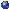## Events

##### Junior GeometryAbhishek Koparde, PMA 12.166: Riemann-Roch and Division Algebras
Tuesday, September 19, 2023, 03:45pm - 04:45pm
The Riemann-Roch theorem provides a delicate control on the number of linearly independent meromorphic functions with prescribed zeros and poles on a Riemann surface. It turns out that this forces the field of meromorphic functions on the Riemann surface to have algebraic properties of great interest. In this talk, we will go through a brief introduction to the Riemann-Roch theorem and division algebras over a field. We then give an exposition of Tsen's theorem which implies there are no nontrivial division algebras over the function field of an algebraic curve. Time permitting, we will give a brief introduction to the Brauer group and how it obstructs the Hasse principle.Location: PMA 12.166